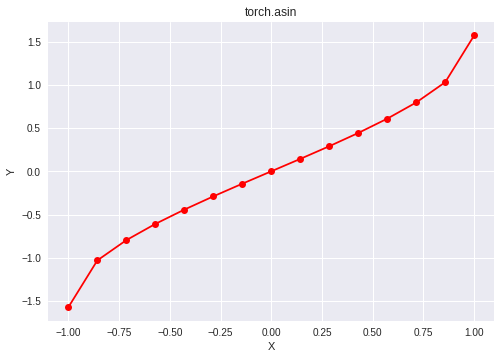# Python | PyTorch asin() method

• Last Updated : 10 Nov, 2021

PyTorch is an open-source machine learning library developed by Facebook. It is used for deep neural network and natural language processing purposes.
The function torch.asin() provides support for the inverse sine function in PyTorch. It expects the input to be in the range [-1, 1] and gives the output in radian form. It returns nan if the input does not lie in the range [-1, 1]. The input type is tensor and if the input contains more than one element, element-wise inverse sine is computed.

Syntax: torch.asin(x, out=None)
Parameters
x: Input tensor
name (optional): Output tensor
Return type: A tensor with the same type as that of x.

Code #1:

## Python3

 `# Importing the PyTorch library` `import` `torch`   `# A constant tensor of size 6` `a ``=` `torch.FloatTensor([``1.0``, ``-``0.5``, ``3.4``, ``0.2``, ``0.0``, ``-``2``])` `print``(a)`   `# Applying the inverse sin function and` `# storing the result in 'b'` `b ``=` `torch.asin(a)` `print``(b)`

Output:

```tensor([ 1.0000, -0.5000,  3.4000,  0.2000,  0.0000, -2.0000])
tensor([ 1.5708, -0.5236,     nan,  0.2014,  0.0000,     nan])```

Code #2: Visualization

## Python3

 `# Importing the PyTorch library` `import` `torch`   `# Importing the NumPy library` `import` `numpy as np`   `# Importing the matplotlib.pyplot function` `import` `matplotlib.pyplot as plt`   `# A vector of size 15 with values from -1 to 1` `a ``=` `np.linspace(``-``1``, ``1``, ``15``)`   `# Applying the inverse sine function and` `# storing the result in 'b'` `b ``=` `torch.asin(torch.FloatTensor(a))`   `print``(b)`   `# Plotting` `plt.plot(a, b.numpy(), color ``=` `'red'``, marker ``=` `"o"``) ` `plt.title(``"torch.asin"``) ` `plt.xlabel(``"X"``) ` `plt.ylabel(``"Y"``) `   `plt.show()`

Output:

```tensor([-1.5708, -1.0297, -0.7956, -0.6082, -0.4429, -0.2898, -0.1433,  0.0000,
0.1433,  0.2898,  0.4429,  0.6082,  0.7956,  1.0297,  1.5708])```My Personal Notes arrow_drop_up
Recommended Articles
Page :